Python 2: Names, Functions, and Frames

Download this notebook and run it interactively to play with the code yourself! See instructions here

Today, you’ll learn:

1. Names: values and functions
2. Visualizing Function calls
3. Data Types
4. Booleans

Let’s Go!1. Review

Reminder: two options for running a cell.

1. Button
• click on the cell
• click the play button ▶ on the left
2. Keyboard
• click on the cell
• press down “SHIFT” key and then simulataneously tap the “ENTER/RETURN” key. Let go.

In general, as you go through the notebook, run all the code cells even if I don't explicitly tell you to do so

Also, just a reminder. If the last line of a cell is a value, the notebook will display that value for you! Or you can use print.

max(5, 6)
-99
print(max(5,6))
-99
-99
max(5, 6)
variable = 8.9

Nothing was displayed if you run the cell above! That’s because the last line just made a variable. To get something to display then the last line has to be a value.

Sidenote: Tabs

When you write a function, everything in the body of the function has to be tabbed!

• the tabs tell python what’s part of the function and what isn’t

Run the following cell. See how you get an error because of the bad tab in the second function? Note that it says the error is at line 11 which is actually wrong. So when you get an error message, the line number it gives you isn’t always the source of the error.

Fix another_func so it doesn’t error.

def func(x, y, hello):
print("You are inside a function!")
# more stuff
# blah blah
return x + y * hello

def another_func(x, y, hello):
print("Oops!")
# more stuff
# blah blah
return x**y + hello

number = 67
hello_there = 45
ok = 2

Names

Let’s review names.

You’ve learned about two types of names:

1. Variable names
• example: x = 5. x is a variable name
2. Function names
• abs(-5). abs is a function name
def func(x, y, cat):
print("You are inside a function!")
# blah blah
return x + y * cat

number = 6.7
hello_there = "heyyy"
ok = True

The above code creates 4 names:

1. func
2. number
3. hello_there
4. ok

You can visualize with a diagram!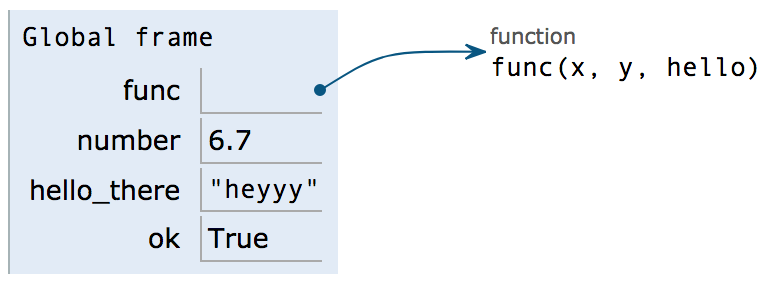As you can see, function names are drawn with an arrow pointing to the function header.

print(func)
print(number)
print(hello_there)
print(ok)

Notice that printing func looks a little funk-y ;)

<function func at 0xMumboJumpo>. It basically means, python knows the name func refers to a function.

What if we do this??

func = 56
print(func)

Now, func no longer refers to a function, but to the number 56.

Oops! We NO LONGER have access to the the function that func once pointed to!

So try to use different names for all your stuff.

Calling Functions

What do you think this function does?

def body_mass_index(height, weight):
return weight / (height ** 2)

The function header requests two arguments: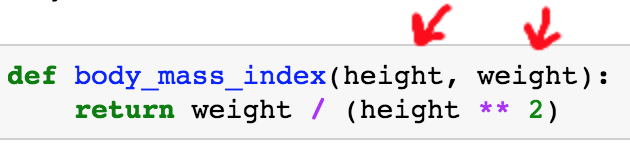<font size=7px>So…</font> When calling the function I need to pass in two values! The values I pass in will then be assigned to height and weight.

# Run me!
body_mass_index(2, 70)

Again, I’ll repeat: The values you pass in will then be assigned to the variables in the function header.Once we call the function body_mass_index, imagine that you suddenly jump up and into the function! Now, height is 2 and weight is 70. We can do the calculations and then return our calculation.

<font size=6px>.. wait… what is ‘return’?</font>

Return literally returns everything to the right of the return statement back to where you called the function! You can imagine erasing your function call and replacing it with the return value: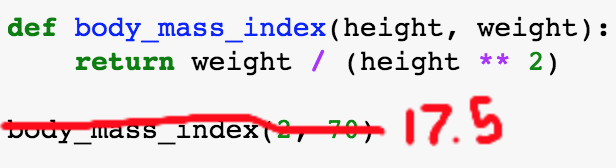We can do stuff with that return value! For example, print it out, or assign a name to it.

fake_bmi = body_mass_index(2, 100) + 5

Just a reminder, to print out my_bmi we have to call print or put it on the last line of a cell!

# last line is below me ;)
fake_bmi
# Call the function again yourself!!

Frames

Okay… so when we were inside the body_mass_index function we were dealing with two names:

1. height
2. weight

However, since those names were made in the function header (in the argument list), we can only access them inside the body_mass_index function.

Will the following cell then error? Run it!

def body_mass_index(height, weight):
return weight / (height ** 2)

print(body_mass_index(1, 10))

print(body_mass_index(1, 34))

print(weight * 2)

The error message says name 'weight' is not defined. That’s because outside of the body_mass_index function it’s true - we haven’t defined weight.

<font size=5px>We could do this … </font>

weight = 60

def body_mass_index(height, weight):
return weight / (height ** 2)

print(body_mass_index(1, 10))

print(body_mass_index(1, 34))

print(weight * 2)

What’s happening here is that there are two versions of weight. There’s the “global” weight which equals 60.

But when we enter body_mass_index we redefine weight as 10 - just for that function call!

For the second function call, weight=34

But once we are outside of the function, we go back to using the ‘global’ version of weight which is 60.

<font size=7px>Whew!!</font> If this last point was a little weird, don’t worry too much about it. Normally you can just use different variable names.

Java Visualizer

However, it is useful to keep track of our names and which names we have access to. Clearly, when you're inside the `body_mass_index` function I have access to different names than when I'm outside.

Every time we call a function, we create a new ‘frame’. Each frame gives us specific access to names. When we return from a function, we exit the frame.

You can diagram this manually, or use a handy python visualizer tool

Click the link, copy the code below into the box and click “visualize execution”.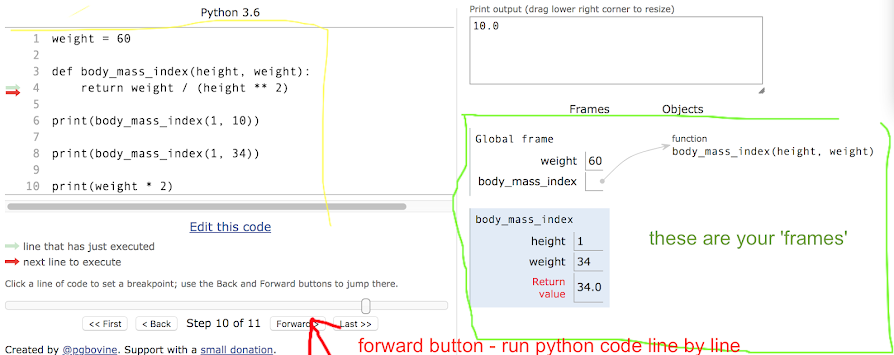Run the visualizer and run the program slowly, line by line by clicking ‘forward’. Look at the frames and see what is happening.

Another example

Let’s look at the familiar code below. Earlier we said we have 4 names.

But what about x, y, and cat?

Once we call func, we are inside the func function and we also have access to x, y, and cat. Being inside the function is called being inside the func frame!! If we aren’t inside any function then we are part of the “global frame”.

Copy and paste the following code into Python Visualizer

def func(x, y, cat):
print("You are inside a function!")
# blah blah
return x + y * cat

number = 6.7
hello_there = 5
ok = 2
func(number, hello_there, ok)

Data Types

We’ve hinted this, but just to mention formally. Names can refer to DIFFERENT TYPES OF DATA.

1. Numbers
2. Functions
3. Strings
4. Booleans

Read on to learn about a fourth type!!

Booleans

Booleans are simple, they’re True or False

Note that they are a different from the word “True” or “False”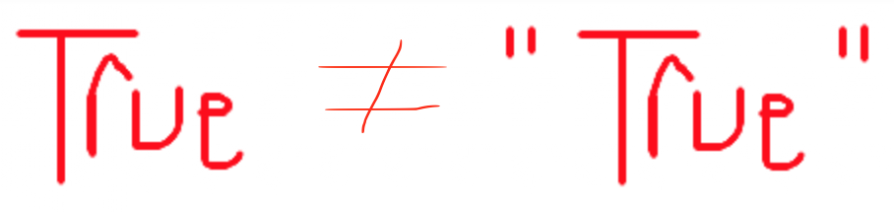Why have booleans? They are useful in if statements.

a = True

if (a):
print("hello Sylvia")
x = 3

statement = x > 5

print(statement)

if (statement):
print("x is greater than 5")

We can use and , or to make more complicated truth statements.

• and : both statements have to be True for the whole thing to be True.

• or : either statement can be True for the whole thing to be True.

and_statement = x > 5 and x < 5
and_statement

While Loops

We can use booleans to execute while loops as well. while loops repeat a section of code until the condition is false.

while (condition):
continue running
coutinue running
x = 1

while (x < 5):
print("Hi for the " + str(x) + " time!")
x = x + 1

Note!! If we did not include the x = x + 1 line then we would be in an infinite loop! Make sure your condition will be False at some point.

Strings : in

You can check if a part of a string is in another string, using the in keyword.

"h" in "hello"
"hello" in "h"
"cap" in "escape"

Exercises

Note: """zippity doo""" are another way of making comments. Often they are used in functions to specify examples of what the function should do!

Fix the Bug

The following snippet of code doesn’t work! Figure out what is wrong and fix the bug.

def both_positive(x, y):
"""Returns True if both x and y are positive.

>>> both_positive(-1, 1)
False
>>> both_positive(1, 1)
True
"""
return x and y > 0 # Replace this line!

Alfonso’s Jacket

Alfonso will only wear a jacket outside if it is below 60 degrees or it is raining. Write a function that takes in the current temperature and a boolean value telling if it is raining and returns True if Alfonso will wear a jacket and False otherwise. First, try solving this problem using an if statement.

def wears_jacket_with_if(temp, raining):
"""
>>> wears_jacket(90, False)
False
>>> wears_jacket(40, False)
True
>>> wears_jacket(100, True)
True
"""
# write your code here and below

Try to write the same function using one line!

def wears_jacket_with_if(temp, raining):
# one line here

Whiling away

Write a function that returns True if n is a prime number and False otherwise.

Hint: use the % operator: x % y returns the remainder of x when divided by y.

def is_prime(n):
"""
>>> is_prime(10)
False
>>> is_prime(7)
True
"""

Try to draw the diagram for the following code.

Remember, we make a new frame when we call a function. Check your solution using the visualizer.

x = 3
def square(x):
return x ** 2
square(2)

Tricky names

Before running the following code, try to think about what the final output will be (what will hmm(wow) return?)

def double(x):
return x * 2

hmmm = double
wow = double(3)
hmmm(wow)

Returning a function

Before running the following code, try to think about what the outputs will be.

See if you understand what’s going on here. I write a function that returns a function :O